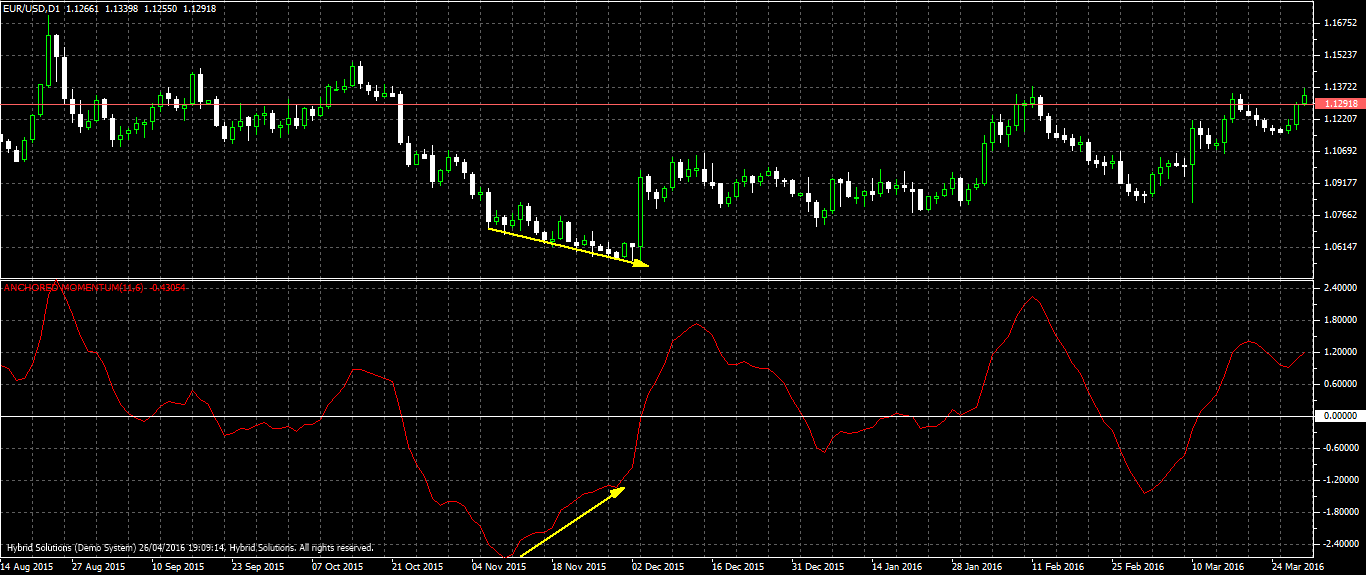# Anchored Momentum

Free

Developed By: JoySebastian

In stock

Anchored Momentum is a VeretexFX Client side modified VTL momentum Oscillator. It is calculated after smoothing the price with moving averages. Thus it anchors the momentum to a moving average, unlike other momentum indicators that anchor the price to n bars back reference point.

SKU: 1097 Categories: ,Anchored Momentum is a VeretexFX Client side modified VTL momentum Oscillator. It is calculated after smoothing the price with moving averages. Thus it anchors the momentum to a moving average, unlike other momentum indicators that anchor the price to n bars back reference point.

It oscillates above and below the zero line. This indicator is mainly used to open buys when Anchored Momentum crosses above the level zero. A reverse situation is suitable for sells; the sells should be opened if the indicator below the level zero. It is better to confirm the signals with other indicators before opening positions.

The zero line cross over may produce many choppy signals. Many such random cross overs can be eliminated by using a buffer zone around the zero line, usually -0.2 to 0.2. Divergence between Anchored Momentum and price is a good signal. When the indicator is rising from the lows below zero level and price is declining to new lows, price reversal is likely to happen immediately. When the indicator line begins to decline above zero level and price is still rising to new highs, there is high probability that the upward price move is losing steam and a reversal is imminent. The parameters in the indicator are momentum calculation period, smoothing period.

Default values for momentum period is 11 and smoothing period is 6. The level at which a horizontal line is to be plotted can be set in the parameter level.